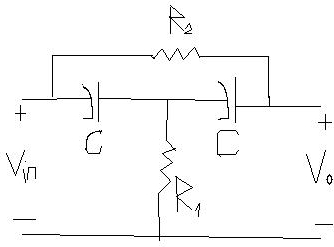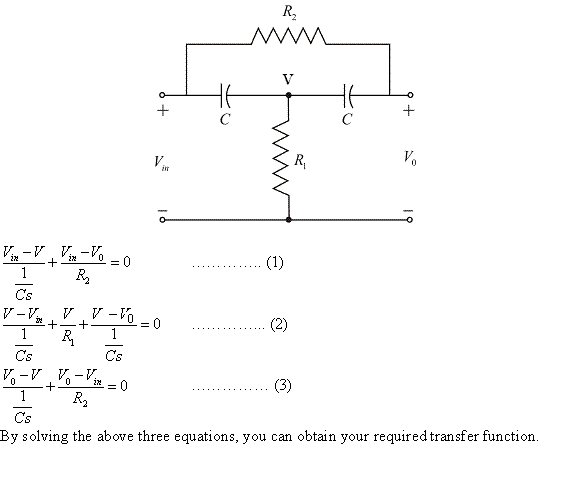Question# A bridges-T network is often used in AC control systems as afilter network.

Other
ANSWEREDA bridges-T network is often used in AC control systems as afilter network. The circuit of one bridged-T network is shown in Figure. Show that the transfer function of the network is;
$$\displaystyle{\frac{{{V}_{{0}}{\left({S}\right)}}}{{{V}_{{\in{\left({S}\right)}}}}}}={\frac{{{1}+{2}{R}_{{1}}{C}_{{\delta}}+{R}_{{1}}{R}_{{2}}{C}^{{2}}{s}^{{2}}}}{{{1}+{\left({2}{R}_{{1}}+{R}_{{2}}\right)}{C}_{{\delta}}+{R}_{{1}}{R}_{{2}}{C}^{{2}}{s}^{{2}}}}}$$
Sketch the pole-zero diagram when $$\displaystyle{R}_{{1}}={0.5},{R}_{{2}}={1}$$ and $$\displaystyle{C}={0.5}$$